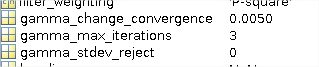# Error PS_EST_GAMMA_QUICK Error using :

I thank if somebody can help me with the following errors when running PS_EST_GAMMA_QUICK

I run several times mt_prep_snap, step 1 and 2 of stamps by using 4 and 9 patches, also deleting all the files generated created from mt_prep_snap process, to start every try with a clear input, but I always get the same error:
Found look angle file
PS_EST_GAMMA_QUICK: n_trial_wraps=0.664205n_trial_wraps=0.650650
PS_EST_GAMMA_QUICK: Initialising random distribution…
Error using :
Colon operands must be real scalars.

Error in ps_topofit (line 31)
trial_mult=[-ceil(8n_trial_wraps):ceil(8n_trial_wraps)]+asym8n_trial_wraps;

Error in ps_est_gamma_quick (line 169)
[K_r,C_r,coh_r]=ps_topofit(exp(j*rand_ifg(i,:)),bperp,n_trial_wraps,‘n’);

Error in stamps (line 326)
ps_est_gamma_quick(est_gamma_parm);

I am using 34 interferograms, with a subset based on 3 bursts (SW1).
I am using the same MATLAB version that with my first try (5 interferograms), and I only installed StaMPS and all the dependences, also MATLAB, in other computer (always Win 10 64 bits and Ubuntu 18.04).
So, I think is not a problem related to the MATLAB.
Maybe a problem with a kind of input containing no real numbers? or with large data?

The first `PST_EST_GAMMA_QUICK:` line gives an odd looking `n_trial_wraps=Xn_trial_wraps=X` entry. Note tat it is much more useful to paste text output as text rather than attaching an image that is unreadable on laptop screens and can’t be searched or indexed.

Thank you very much gnwiii.
I changed the image by the code text. Is there any way to know the approximately or correct values for n_trial_wraps?
Maybe it is related to some erroneous values in my input files, and how can I check it?

Or are you saying that it should give only a value of n_trial_wraps, for example:
PS_EST_GAMMA_QUICK: n_trial_wraps=0.664205
PS_EST_GAMMA_QUICK: Initialising random distribution…

As I found in this conversation:

If n_trial_wraps has to receive only one value, it is very rare. I downloaded the last StaMPS version, which worked fine on my first try (5 interferograms). I have not idea of how compile the MATLAB functions in this case, but I also think that the problem is in that step of passsing the value to n_trial_wraps.

StaMPS Manual page 19 says:

The parameters that control the processing are set to default values which you can view with:
`matlab`
`>>getparm`
You can modify any parameters from the default using
`>>setparm(‘param name’,param value)`
Only enough characters of param name to make it unique are required. Setting param value to nan resets the parameter to the default value.

I understand. However, I don’t see what is the parameter related to n_trial_wraps.
Related with step 2 and the calculation of gamma, i only see gamma_change_convergence, gamma_max_iterations and gamma_stdev_reject. But I don’t know if have any sense to modify some of the parameters in parms.mat.SOLVED: the error ocurred when I tried to process all the patches by launching step 2 from the main folder (INSAR_“MASTER_DATE” folder). Although I didn’t find other parms.mat files within each subfolder (of the patches). Now I generated an unique PATCH_1 on which I run step 2 and the n_trial_wraps has a more logical value.

STAMPS: ########################################
STAMPS: ################ Step 2 ################
STAMPS: ########################################
STAMPS: Directory is PATCH_1

PS_EST_GAMMA_QUICK: Starting
PS_EST_GAMMA_QUICK: Estimating gamma for candidate pixels
GETPARM: filter_grid_size=50
GETPARM: filter_weighting=‘P-square’
GETPARM: clap_win=32
GETPARM: clap_low_pass_wavelength=800
GETPARM: clap_alpha=1
GETPARM: clap_beta=0.3
GETPARM: max_topo_err=20
GETPARM: lambda=0.0554658
GETPARM: gamma_change_convergence=0.005
GETPARM: gamma_max_iterations=3
GETPARM: small_baseline_flag=‘n’
Found look angle file
PS_EST_GAMMA_QUICK: n_trial_wraps=0.258353
PS_EST_GAMMA_QUICK: Initialising random distribution…
PS_EST_GAMMA_QUICK: 1902394 PS candidates to process
PS_EST_GAMMA_QUICK: iteration #1
PS_EST_GAMMA_QUICK: Calculating patch phases…

So, I think, that for large areas with more than one PATCH, it is better to run all steps on each PATCH folder, not from the main folder to process. Although my area doesn’t seem so large, but in the future I can try with 2 PATCHES (for example).

Thank you for your tip anyway!

1 Like

This error continues, at least when I change the parameter max_topo_error.
When I delete all the files created by StaMPS and by the mt_prep_snap function and start clear processing without changing max_topo_erorr, the error doesn’t appear. Thus, I can’t avoid this error when I change this parameter. If somebody gets any interesting feedback about this error, please, let us know.